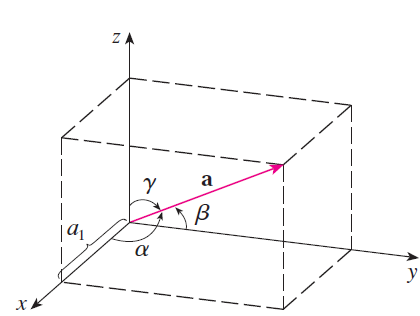# Introduction to Vectors

A vector is a quantity that has a magnitude and a direction. This differs from a scalar quantity that only has a magnitude.

Some examples with unts are shown below:

Name Quantity Vector/Scalar
Speed $$12 \; [\frac{m}{s}]$$ Scalar
Velocity $$25 \; [\frac{m}{s}] \; 110^\circ$$ Vector
Area $$500 \; [cm^2]$$ Scalar
Temperature $$22 \; [^\circ C]$$ Scalar
Force $$45 \; [N] \; 20^\circ \; S \; of \; E$$ Vector
Acceleration $$\left<2, 3, -5\right> [\frac{m}{s^2}]$$ Vector
Distance $$89 \;[mm]$$ Scalar
Displacement $$100 \; [m] \; W$$ Vector

A quantity is described as "10 units upwards". Is this quantity a scalar or a vector?

You can see some quantities are very similar (such as distance and displacement or speed and velocity) but some are vectors. Vectors are important because quantities interact in space. For example, if two athletes start running at the same location but one person runs East and another runs North, even after they both run 1 km (distance) they are nowhere near eachother because there displacements differ (1 km E vs 1 km N). Without the directional information, you might think the runners are next to each other.

We need to understand how vectors interact. Consider a game of tug of war. If both teams exert a force of 100 N, the pulling will cancel out and neither team will be winning. This can be understood by realizing forces are vectors!

## Vector Notation

Vectors are represented as letters with arrows on top such as $$\vec{u}$$. A vector can be made between two points, the initial point (or tail) and terminal point (or head) like $$\vec{AB}$$. In some textbooks, vectors are just bolded like $$\textbf{u}$$ or $$\textbf{AB}$$. Vectors can also be represented by showing the quantities along each direction (x, y, etc) such as $$(-1,2)$$ or $$\left<1,1, -7\right>$$. Scalars are represented as single numbers or letters like $$5$$ or $$u$$.

Sometimes, just the magnitude portion of the vector is required using the notation $$|\vec{u}|$$ or $$||\vec{u}||$$.

What is the magnitude of $$\vec{u}=9 \; [\frac{m}{s}] \; N$$?

What is the magnitude of $$\vec{u} = \left<5, 8, 9, -2, -1\right>$$?

## Drawing Vectors

A vector can be shown as an arrow. The direction of the arrow represents the direction of the vector and the length represents the magnitude. Below, $$\vec{u}$$ has a larger magnitude than $$\vec{v}$$ and a different direction.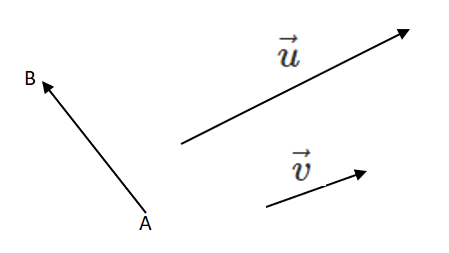The direction portion of a vector can be shown in different ways.

Bearing Angles.
Angle starting at North and rotated clockwise like "the bearing of A from B is $$45^\circ$$".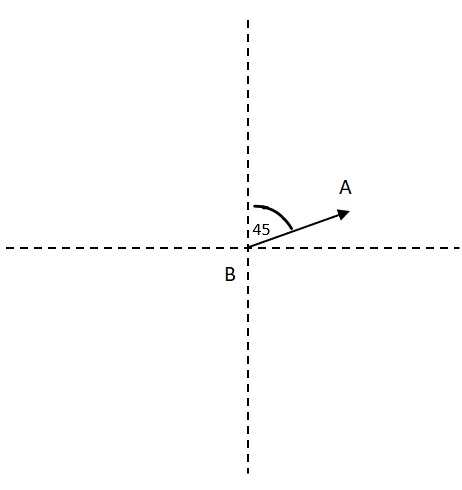Directional Angles
Described as angles from North, East, South, West like "$$20^\circ \; S \; of \; W$$".Math Angles
Described as angles counter-clockwise from the positive x-direction like "$$120^\circ$$".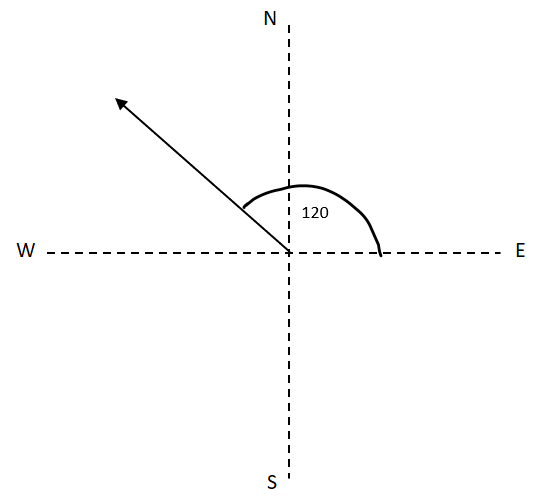Components
Described as components along each direction like "$$\left<5, -3\right>$$" or "$$5\hat \imath + -3\hat \jmath$$".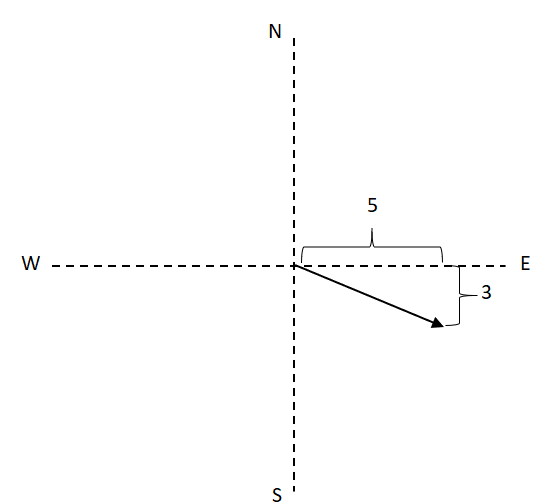##### Try these questions:

The first three types of vectors are Geometric Vectors (magnitude and direction) and the fourth is an Algebraic Vector (components). We will learn about different strategies to work with geometric and algebraic vectors.

To convert a Geometric Vector to an Algebraic Vector we need to find the magnitude of the components in each direction. In 2-D space, use SOH CAH TOA:

$$|\vec{a}| = 20, \theta = -30^\circ$$

$$a_x = 20cos(30^\circ) = 17.32$$

$$a_y = -20sin(30^\circ) = -10$$

$$\vec{a} = \left< 17.32, -10 \right>$$In 3-D, use cosine angles:

$$|\vec{a}| = 20, \alpha = 60^\circ, \beta = 30^\circ, \gamma = 40^\circ$$

$$a_x = 20cos(60^\circ) = 10$$

$$a_y = 20cos(30^\circ) = 17.32$$

$$a_z = 20cos(40^\circ) = 15.32$$

$$\vec{a} = \left<10, 17.32, 15.32\right>$$